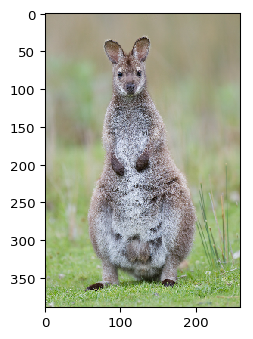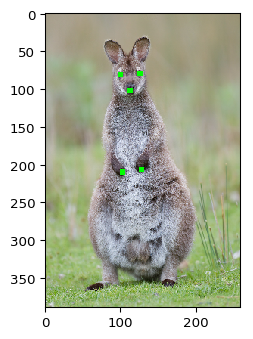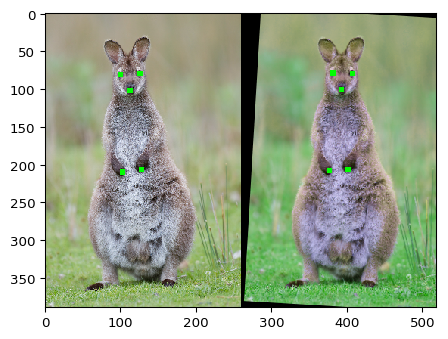# Augment Keypoints/Landmarks¶

Keypoints in imgaug are points on images, given as absolute x- and y- pixel coordinates with subpixel accuracy (i.e. as floats with value range [0, S), where S denotes the size of an axis). In the literature they are also called "landmarks" and may be used for e.g. human pose estimation.

In imgaug, keypoints are only affected by augmenters changing the geometry of images. This is the case for e.g. horizontal flips or affine transformations. They are not affected by other methods, such as gaussian noise.

Two classes are provided for keypoint augmentation in imgaug, listed in the following sections.

## API: Keypoint¶

imgaug.augmentables.kps.Keypoint: A very simple class instantiated as Keypoint(x=<number>, y=<number>). Noteworthy methods of the class are:

• project(from_shape, to_shape): Used to project keypoints from one image shape to another one, e.g. after image resizing.
• shift(x=0, y=0): Used to move points on the x/y-axis. Returns a new Keypoint.
• draw_on_image(image, color=(0, 255, 0), alpha=1.0, size=3, copy=True, raise_if_out_of_image=False): Draw this keypoint on an image.

## API: KeypointsOnImage¶

imgaug.augmentables.kps.KeypointsOnImage: Combines a list of keypoints with an image shape. It is instantiated as KeypointsOnImage(keypoints, shape), where keypoints is a list of Keypoint instances and shape is the shape of the image on which the keypoints are placed. Both arguments are later available as .keypoints and .shape attributes. Noteworthy methods are:

• on(image): Used to project the keypoints onto a new image, e.g. after image resizing.
• draw_on_image(image, color=(0, 255, 0), alpha=1.0, size=3, copy=True, raise_if_out_of_image=False): Draw keypoints as squares onto an image. The image must be given as a numpy array.
• shift(x=0, y=0): Analogous to the method in Keypoint, but shifts all Keypoints in .keypoints.
• to_xy_array(): Transforms the instance to a (N, 2) numpy array.
• from_xy_array(xy, shape): Creates an instance of KeypointsOnImage from an (N, 2) array. shape is the shape of the corresponding image.
• to_distance_maps(inverted=False): Converts the keypoints to (euclidean) distance maps in the size of the image. Result is of shape (H, W, N), with N being the number of keypoints.
• from_distance_maps(distance_maps, inverted=False, if_not_found_coords={"x": -1, "y": -1}, threshold=None, nb_channels=None): Inverse function for to_distance_maps().

## API: Methods¶

To augment keypoints, the method augment(images=..., keypoints=...) may be called. An alternative is augment_keypoints(), which only handles keypoint data and expects a single instance of KeypointsOnImage or a list of that class.

## Basic example¶

Let's take a look at a simple example, in which we augment an image and five keypoints on it by applying an affine transformation. As the first step, we load an example image from the web:

In :
import imageio
import imgaug as ia
%matplotlib inline

image = ia.imresize_single_image(image, (389, 259))
ia.imshow(image)Now let's place and visualize a few keypoints:

In :
from imgaug.augmentables.kps import Keypoint, KeypointsOnImage
kps = [
Keypoint(x=99, y=81),   # left eye (from camera perspective)
Keypoint(x=125, y=80),  # right eye
Keypoint(x=112, y=102), # nose
Keypoint(x=102, y=210), # left paw
Keypoint(x=127, y=207)  # right paw
]
kpsoi = KeypointsOnImage(kps, shape=image.shape)

ia.imshow(kpsoi.draw_on_image(image, size=7))Note how we "merged" all keypoints of the image in an instance of KeypointsOnImage. We will soon augment that instance. In case you have to process the keypoints after augmentation, they can be accesses via the .keypoints attribute:

In :
print(kpsoi.keypoints)

[Keypoint(x=99.00000000, y=81.00000000), Keypoint(x=125.00000000, y=80.00000000), Keypoint(x=112.00000000, y=102.00000000), Keypoint(x=102.00000000, y=210.00000000), Keypoint(x=127.00000000, y=207.00000000)]


Now to the actual augmentation. We want to apply an affine transformation, which will alter both the image and the keypoints. We choose a bit of translation and rotation as our transformation. Additionally, we add a bit of color jittering to the mix. That color jitter is only going to affect the image, not the keypoints.

In :
import imgaug.augmenters as iaa
ia.seed(3)

seq = iaa.Sequential([
iaa.Affine(translate_px={"x": (10, 30)}, rotate=(-10, 10)),
iaa.AddToHueAndSaturation((-50, 50))  # color jitter, only affects the image
])


And now we apply our augmentation sequence to both the image and the keypoints. We can do this by calling seq.augment(...) or its shortcut seq(...):

In :
image_aug, kpsoi_aug = seq(image=image, keypoints=kpsoi)


If you have more than one image, you have to use images=... instead of image=.... You will also have to provide a list as input to keypoints. Though that list does not necessarily have to contain KeypointsOnImage instances. You may also provide (per image) a list of Keypoint instances or a list of (x,y) tuples or an (N,2) numpy array.

Make sure however to provide both images and keypoints in a single call to augment(). Calling it two times -- once with the images as argument and once with the keypoints -- will lead to different sampled random values for the two datatypes.

Now let's visualize the image and keypoints before/after augmentation:

In :
import numpy as np
ia.imshow(
np.hstack([
kpsoi.draw_on_image(image, size=7),
kpsoi_aug.draw_on_image(image_aug, size=7)
])
)## Projecting Keypoints onto other Images¶

When working with keypoints, you might at some point have to change the image size. The method KeypointsOnImage.on(image or shape) can be used to recompute keypoint coordinates after changing the image size. It projects the keypoints onto the same relative positions on a new image. In the following code block, the initial example image is increased to twice the original size. Then (1st) the keypoints are drawn and visualized on the original image, (2nd) drawn and visualized on the resized image without using on() and (3rd) drawn and visualized in combination with on().

In :
image_larger = ia.imresize_single_image(image, 2.0)

print("Small image %s with keypoints optimized for the size:" % (image.shape,))
ia.imshow(kpsoi.draw_on_image(image, size=7))

print("Large image %s with keypoints optimized for the small image size:" % (image_larger.shape,))
ia.imshow(kpsoi.draw_on_image(image_larger, size=7))

print("Large image %s with keypoints projected onto that size:" % (image_larger.shape,))
ia.imshow(kpsoi.on(image_larger).draw_on_image(image_larger, size=7))

Small image (389, 259, 3) with keypoints optimized for the size:Large image (778, 518, 3) with keypoints optimized for the small image size: